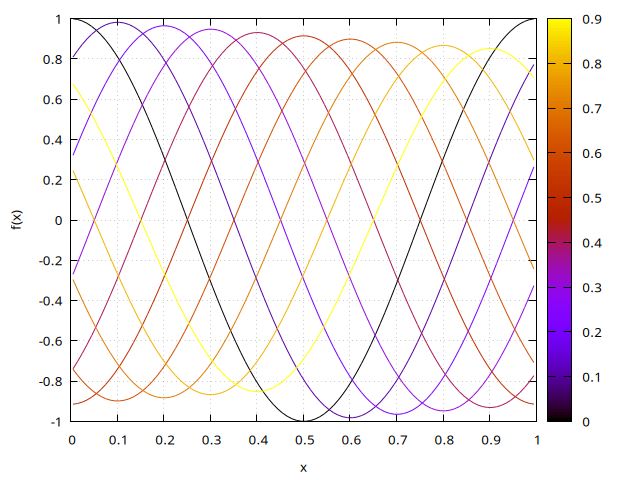# Advection of a cos function (problem with periodic boundary conditions)

This is specific to cartesian1D.h, a simple workaround is to replace this with multigrid1D.h

# include "grid/cartesian1D.h"
#include "utils.h"

void flux_upwind (scalar f, scalar df, double dt)
{
foreach()
boundary ({df});
}

int main() {
int n = 100;
double CFL = 0.1;
double t = 0., tend = 1.;

init_grid (n);

periodic (right);

scalar f[], df[];
foreach() {
f[] = cos(2.*M_PI*x);
}
boundary ({f});

FILE * fp    = fopen ("function.dat", "w");

for (int i = 0; t <= tend; i++) {

// we obtain the flux
flux_upwind (f, df, dt);

// numerical solution at t+dt
foreach()
f[] -= (df[]-df[-1])/Delta;
boundary ({f});

if (i % 100 == 0) {
foreach ()
fprintf(fp, "%g %g %g \n", t, x, f[]);
fprintf(fp, " \n");
}

t += dt;
}

fclose(fp);

free_grid();
}

Temporal evolution

set output 'function.png'
set xlabel 'x'
set ylabel 'f(x)'
set key left
set grid
p "function.dat" u 2:3:1 not w l palette(script)